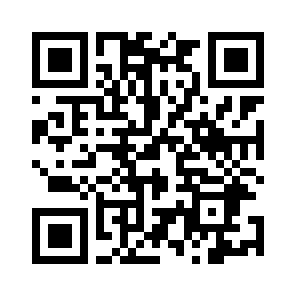# Area and Volume Calculator( 0 )

دسته : آموزش

قیمت : رایگان

نسخه : 5.0

حجم : 475.52 kB

475.52 kB

دانلود : 100,000+

10+

اندروید مورد نیاز : 3.0.x

3.0.x

This free app is a geometric calculator which is able to calculate area and volume.

Area Calculator: You are able to calculate the area for the most important geometric figures. You can calculate the area of circle, ellipse, rectangle, square, trapezoid, triangle, parallelogram, rhombus, sector and triangle.

Volume Calculator: You are able to calculate the volume for the most important geometric objects. You can calculate the volume of cone, cube, cuboid, cylinder, sphere, square pyramid and tetrahedron.

Best math tool for school and college! If you are a student, it will help you to learn geometry!

 باز کردن سوکت های شبکه دسترسی به اطلاعات مربوط به شبکه ها را می دهد.